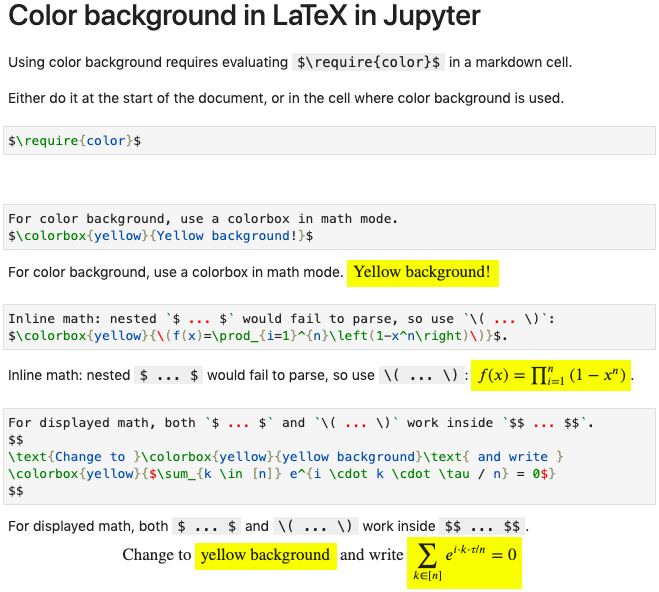# Revision history [back]

Jupyter uses MathJax to render LaTeX. The reference for this question is therefore:

One solution indicated there involves modifying "the load array of the loader block of your MathJax configuration". I did not try that.

The other one, which I tried with success, consists in fist executing a markdown cell with

$\require{color}$


and then using \colorbox, for example:

$\colorbox{yellow}{Writing on yellow background!}$


This enables math fragments on color background, as part of displayed equations.

For instance:

$$\text{Change to }\colorbox{yellow}{yellow background}\text{ and write } \colorbox{yellow}{\sum_{k=1}^n e^{i \cdot k \cdot \tau / n} = 0}$$


Not sure obtaining inline math on colored background is doable.

Jupyter uses MathJax to render LaTeX. The reference for this question is therefore:

One solution indicated there involves modifying "the load array of the loader block of your MathJax configuration". I did not try that.

The other one, which I tried with success, consists in fist executing a markdown cell with

$\require{color}$


and then using \colorbox, for example:

$\colorbox{yellow}{Writing on yellow background!}$


This enables math fragments on color background, as part of displayed equations.

For instance:

$$\text{Change to }\colorbox{yellow}{yellow background}\text{ and write } \colorbox{yellow}{\sum_{k=1}^n e^{i \cdot k \cdot \tau / n} = 0}$$


Not sure obtaining For inline math on colored background math, $\colorbox{yellow}{$ ... $}$ would not parse.

The trick is doable.

to use $\colorbox{yellow}{$...$}$ instead.

In the screenshot below, relevant markdown cells are duplicated, and showed once in edit mode and once in displayed mode.